Question

determine the terminal symbols and non-terminal symbols and the start symbols from the following grammar:

<program> ==> <stmts>

<stmts> ==> <stmt> | <stmt> ; <stmts>

<var> ==> a | b | c | d

<expr> ==> <term>+<term>|<term>-<trerm>

<term> == > <var>|const

Terminal symbol :- | , ; , + , -

Non terminal symbol :- program , stmts, stmt, var ,expr , term

Start symbol :- var

#### Earn Coins

Coins can be redeemed for fabulous gifts.

Similar Homework Help Questions
• ### Consider the following grammar (G1) for simple assignment statements. (The symbols in double quotation marks are...

Consider the following grammar (G1) for simple assignment statements. (The symbols in double quotation marks are terminal symbols.) assign → id “ = ” expr id → “A” | “B” | “C” expr → expr “ + ” expr | expr “ ∗ ” expr | “(” expr “)” | id a) Give a (leftmost) derivation for string A = B ∗ A + C. b) Give the parse tree for string A = B ∗ A + C. c)...

• ### Need answer along with detailed explanation: Which of the following are terminal symbols in the grammar...

Need answer along with detailed explanation: Which of the following are terminal symbols in the grammar rule: <term> -> <term> * <factor> | (<term>) | <term> (May contain more than one possible answer) A.)   | B.)   ( C.)   <term> D.)   *

• ### Consider the following grammar (S, A, B, and C are nonterminal symbols; S is the start...

Consider the following grammar (S, A, B, and C are nonterminal symbols; S is the start symbol; 0 and 1 are terminal symbols): S → AA A → BCB B → B0 | B1 | 0 | 1 C → 00 | 11 Which of the following sentences are in the language generated by the grammar? Show derivations for the sentences that can be generated. If a sentence cannot be generated by the grammar, explain why. a) 10010001 b) 01101101...

• ### 1 Prog-DCs Strnts \$ Terminal Regular Expression floatdcl intdcl I intdcl id print "p" id 6...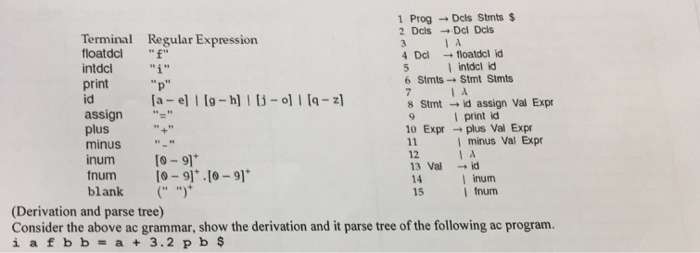1 Prog-DCs Strnts \$ Terminal Regular Expression floatdcl intdcl I intdcl id print "p" id 6 Stmts- Stmt Simts 8 Strnt →id assign Val Expr 10 Expr → plus Val Expr assign " plus minus inum [0-91 fnum 0-9.0-91 blank ("" 2 I print id I minus Val Expr 12 13 Vai -id 14 15 I inum I fnum (Derivation and parse tree) Consider the above ac grammar, show the derivation and it parse tree of the following ac program

• ### Name: 3. (10 points) Given grammar: <program> → <stmts> Page: 2 <term> → <var> 1 const...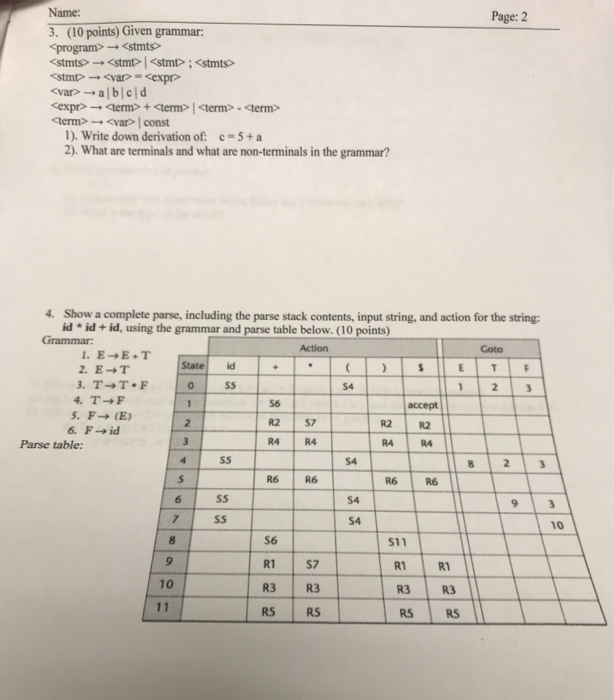Name: 3. (10 points) Given grammar: <program> → <stmts> Page: 2 <term> → <var> 1 const 1), write down derivation of: c-5+a 2) What are terminals and what are non-terminals in the grammar? Show a complete parse, including the parse stack contents, input string, and action for the string: id - id + id, using the grammar and parse table below. (10 points) 4. Grammar State id S4 4. T F 5. F (E) R2 S7 R4 R4 R2İR2 Parse...

• ### 16. A grammar can be expressed in BNF notation. There are two types of symbols in...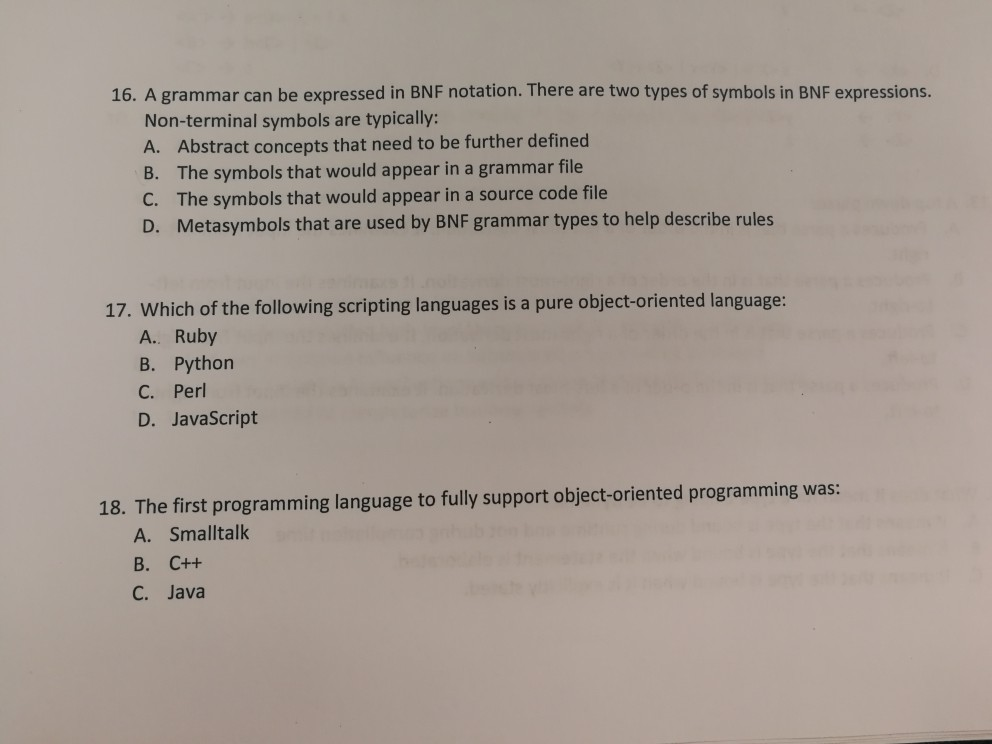16. A grammar can be expressed in BNF notation. There are two types of symbols in BNF expressions. Non-terminal symbols are typically A. Abstract concepts that need to be further defined B. The symbols that would appear in a grammar file C. The symbols that would appear in a source code file D. Metasymbols that are used by BNF grammar types to help describe rules 17. Which of the following scripting languages is a pure object-oriented language: A.. Ruby B....

• ### Using the grammar below: <program> → begin <stmt_list> end <stmt_list> <stmt> | <stmt>; <stmt_list> <stmt> <var>...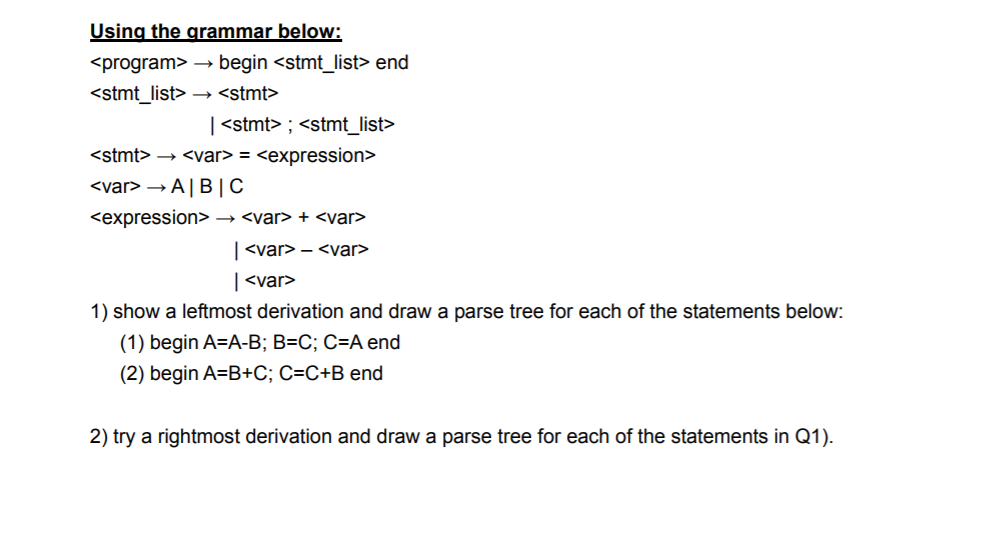Using the grammar below: <program> → begin <stmt_list> end <stmt_list> <stmt> | <stmt>; <stmt_list> <stmt> <var> = <expression> <var> → ABC <expression> <var> + <var> | <var> - <var> | <var> 1) show a leftmost derivation and draw a parse tree for each of the statements below: (1) begin A=A-B; B=C; C=A end (2) begin A=B+C; C=C+B end 2) try a rightmost derivation and draw a parse tree for each of the statements in Q1).

• ### Consider the following grammar: (//some alternative rules are listed on separate lines without using symbol |):...

Consider the following grammar: (//some alternative rules are listed on separate lines without using symbol |): stmt −> assignment −> subr call assignment −> id := expr subr call −> id ( arg list ) expr −> primary expr tail expr tail −> op expr −> ε primary −> id −> subr call −> ( expr ) op −> + | - | * | / arg list −> expr args tail args tail −> , arg list −> ε...

• ### Question 3: Given the following grammar: assign → id := expr expr → expr + term \ term term -term *factor lfactor factor-(expr) id Using the above grammar, show a leftmost derivation (first five step...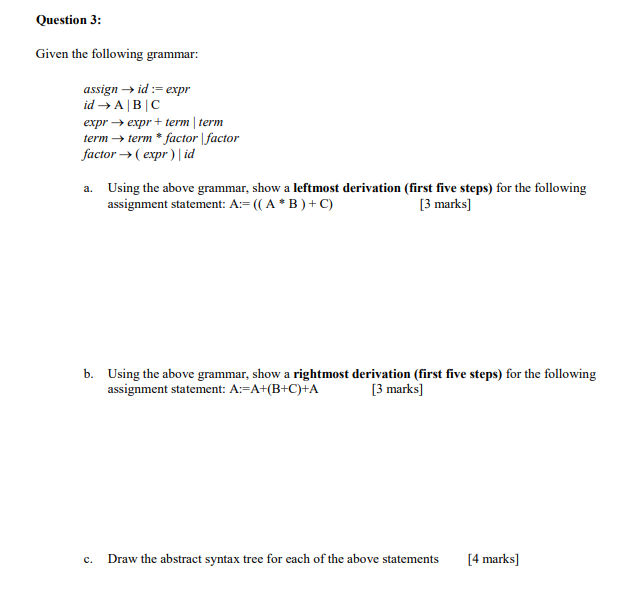Question 3: Given the following grammar: assign → id := expr expr → expr + term \ term term -term *factor lfactor factor-(expr) id Using the above grammar, show a leftmost derivation (first five steps) for the following assignment statement: A ((A B)+ C) a. [3 marks] b. Using the above grammar, show a rightmost derivation (first five steps) for the following assignment statement: A:-A+B+C)+A [3 marks] Draw the abstract syntax tree for each of the above statements [4 marks]...

• ### The questions in this section are based on the grammar given as the following: prog ->...

The questions in this section are based on the grammar given as the following: prog -> assign | expr assign -> id = expr expr -> expr + term | expr - term | term term -> factor | factor * term factor -> ( expr ) | id | num id -> A | B | C num -> 0 | 1 | 2 | 3 (2a) What is the associativity of the * operator? (5 points) (2b) What...